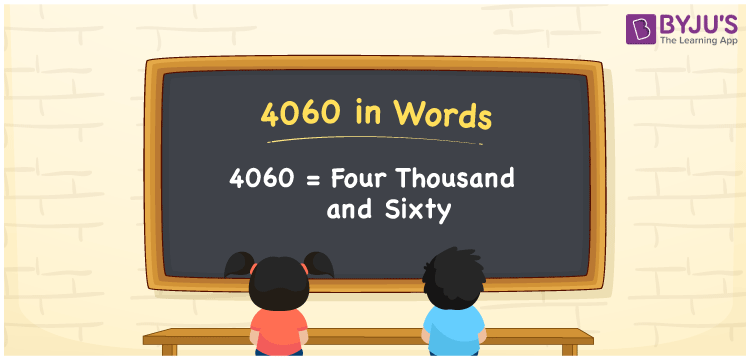# 4060 in Words

The spelling of 4060 in English words is “four thousand and sixty”. The number 4060 contains 4 digits and it lies between 4059 and 4061. Also, the numeral 4060 is a cardinal number. For example, if you have earned Rs. 4060 in two months, you can write it as “I have earned Rs. Four thousand and sixty in two months”.

 4060 in Words: Four Thousand and Sixty. Four Thousand and Sixty in Numerical Form: 4060.

## 4060 in English Words## How to Write 4060 in Words?

Understand the place values of 4060 using the below table and convert the number 4060 into words.

 Thousands Hundreds Tens Ones 4 0 6 0

The expanded form of 4060 is as follows:

= 4 × Thousand + 0 × Hundred + 6 × Ten + 0 × One

= 4 × 1000 + 0 × 100 + 6 × 10 + 0 × 1

= 4000 + 60

= 4060

= Four thousand and sixty

Hence, 4060 in words is four thousand and sixty.

4060 in words – Four thousand and sixty

Is 4060 an odd number? – No

Is 4060 an even number? – Yes

Is 4060 a perfect square number? – No

Is 4060 a perfect cube number? – No

Is 4060 a prime number? – No

Is 4060 a composite number? – Yes

## Frequently Asked Questions on 4060 in Words

Q1

### How to spell 4060?

4060 in words is four thousand and sixty.

Q2

### Simplify 4000 + 60, and express it in words.

Simplifying 4000 + 60, we get 4060. Hence, 4060 in words is four thousand and sixty.

Q3

### Is 4060 an even number?

Yes, 4060 is an even number.